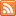# Content Tagged “Probability of At Least One”## Basic Probability Rules

Published: Sep 19th, 2013

View Lecture Slides with Transcript – Basic Probability Rules Part 1 (12:51) Slides 1-11 Part 2 (12:26) Slides 12-20 This document is linked from Basic Probability Rules.

## Interactive Applet: Probability Venn Diagram

Published: Aug 18th, 2013

Now that we have introduced most of the basic probability concepts, try these interactive demonstration which uses Venn diagrams to illustrate the basic probabilities we have been discussing. You can choose […]## Basic Probability Rules

Published: Jul 28th, 2012

Introduction Rules of Probability Probability Rule One (For any event A, 0 ≤ P(A) ≤ 1) Probability Rule Two (The sum of the probabilities of all possible outcomes is 1) Probability Rule […]